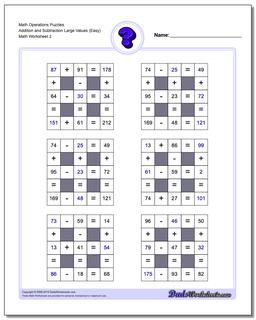One Dad. Four daughters. 9,268 worksheets... and counting!# Math Operations Puzzles Addition and Subtraction Large Values (Easy) (Second Worksheet)

PropertyValue
DescriptionMath Operations Puzzles Addition and Subtraction Large Values (Easy): Math logic puzzle worksheets that require students to fill in missing values. These worksheets have larger values for the operands and the sums than the ones above, and are appropriate for 4th grade students comfortable with larger problems. (Second Worksheet)
Resource TypeWorksheet